# AP Physics 2 : Bernoulli's Equation

## Example Questions

← Previous 1 2 Next →

### Example Question #11 : Bernoulli's Equation

Water is flowing through a hose. It comes out of the tap at a pressure of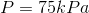, velocity of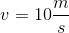, and height of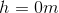. It leaves the nozzle at a pressure of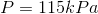and a height of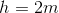. What is the velocity of the water when it leaves the hose?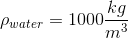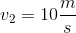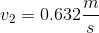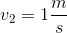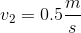Explanation:

To solve this problem, we will use Bernoulli's equation, a simplified form of the law of conservation of energy. It applies to fluids that are incompressible (constant density) and non-viscous.

Bernoulli's equation is: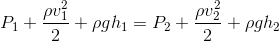Whereis pressure,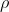is density,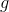is the gravitational constant,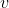is velocity, andis the height.

In our question, state 1 is at the tap and state 2 is at the nozzle. Input the variables from the question into Bernoulli's equation: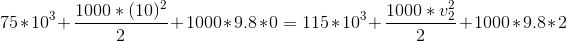Simplify and solve for the final velocity.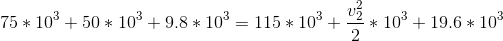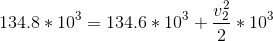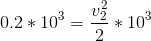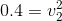### Example Question #12 : Bernoulli's Equation

Water is flowing through a horizontal pipe. Water enters the left side of the pipe at a pressure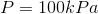and a velocity of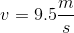. It leaves the pipe at a velocity of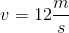. What is the pressure of the water when it leaves the pipe?.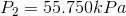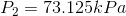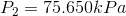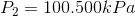Explanation:

To solve this problem, we will use Bernoulli's equation, a simplified form of the law of conservation of energy. It applies to fluids that are incompressible (constant density) and non-viscous.

Bernoulli's equation is:Whereis pressure,is density,is the gravitational constant,is velocity, andis the height. In our question, state 1 is at the entrance of the pipe and state 2 is at the exit of the pipe. Both states of the pipe are at the same height because the pipe is horizontal. Input the variables from the question into Bernoulli's equation and solve for the final pressure: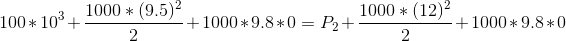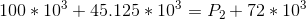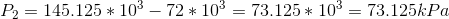### Example Question #11 : Bernoulli's Equation

Water is flowing through a horizontal pipe. Water enters the left side of the pipe at a pressureand a velocity of. It leaves the pipe at a pressure of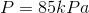. What is the velocity of the water when it leaves the pipe?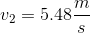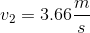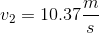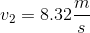Explanation:

To solve this problem, we will use Bernoulli's equation, a simplified form of the law of conservation of energy. It applies to fluids that are incompressible (constant density) and non-viscous.

Bernoulli's equation is:Whereis pressure,is density,is the gravitational constant,is velocity, andis the height.

In our question, state 1 is at the entrance of the pipe and state 2 is at the exit of the pipe. Both states of the pipe are at the same height because the pipe is horizontal. Input the variables from the question into Bernoulli's equation and solve for the final velocity: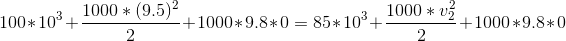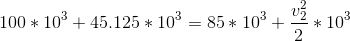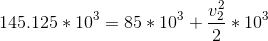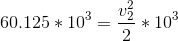### Example Question #14 : Bernoulli's Equation

As the velocity of a fluid increases, what happens to the pressure? Assume both states of the fluid are at the same height and the pipe has a constant diameter.

Velocity has no effect on pressure

As velocity increases, the pressure decreases

As velocity increases, the pressure stays the same

As velocity increases, the pressure increases

As velocity increases, the pressure decreases

Explanation:

To solve this problem, we will use Bernoulli's equation, a simplified form of the law of conservation of energy. It applies to fluids that are incompressible (constant density) and non-viscous.

Bernoulli's equation is:Whereis pressure,is density,is the gravitational constant,is velocity, andis the height. Since the height of the pipe is constant, we can eliminate the height term leaving us with: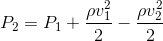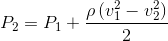The velocity is increasing,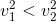making the velocity term negative. We are subtracting some amount from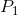, therefore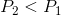, meaning the pressure is decreasing.

### Example Question #51 : Fluid Dynamics

Suppose a physician is considering the flow of blood through a blood vessel. Dissolved within the blood are several important gasses, such as oxygen. If there is a blood clot that is partially obstructing one of the blood vessels, how will the blood's partial pressure of oxygen change as it is flowing past this clot?

It will not change

It will decrease

There is no way to predict how it will change

It will increase

It depends on the amount of blood in circulation

It will decrease

Explanation:

In this question, we're presented with a scenario in which blood is flowing through a blood vessel with a clot. We're asked to determine how the partial pressure of oxygen changes as the blood flows pass the clot. To answer this, we'll need to understand the concepts of the continuity equation, Bernoulli's equation, and partial pressures.

Since the blood is flowing past an obstruction, and because the volume flow rate is the same everywhere, we can use the continuity equation to show the relationship between velocity and area.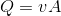What this equation shows is that, when the volume flow rate is constant, the velocity of the fluid is inversely proportional to the area in which it is flowing. When the blood passes through the narrow space caused by the obstruction, it temporarily speeds up and thus has a higher velocity.

Next, we can use the Bernoulli equation to show how the velocity is related to pressure.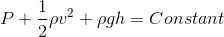In this case, we can assume that the height does not change, and thus we can take the potential energy component out of the equation. What we are left with is an inverse relationship between velocity and pressure. Thus, as the velocity increases, the pressure decreases.

The next thing to do is relate total pressure with partial pressure. Remember that partial pressure refers to the proportion of pressure that a certain gas contributes to the overall pressure when there is a mixture of gasses. For instance, the partial pressure of oxygen in the atmosphere is roughly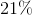, meaning that oxygen is responsible for contributingof the overall pressure of the atmosphere. The expression for partial pressure is as follows.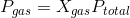Again, what this means is that the partial pressure of a certain gas is dependent on both the fraction of that gas out of all other gasses present, and also the total pressure. Even though the proportion of oxygen will remain the same as blood flows pass the clot, the total pressure in that region decreases. Thus, the partial pressure of oxygen in this region will decrease as well.

All in all, let's summarize what happened by bringing it all together. As the blood flows through a narrower space due to the obstruction, its velocity increases. As a result of the increase in velocity, the pressure lessens. And as the pressure decreases, so too does the partial pressure of any of the gasses within that region, including that of oxygen.

← Previous 1 2 Next →

### All AP Physics 2 Resources